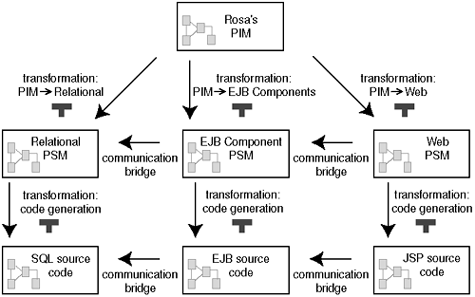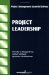# 4.2 Applying the MDA Framework

Rosa will be interested only in the final system. But, because this is an example of how the MDA framework can be applied, we are interested in the process of building Rosa's Breakfast System. We will dissect this process into parts that have meaning within the MDA framework. We must identify which PSMs and code models should be delivered and which transformation definitions should be used to generate the PSMs and code models. All elements of the MDA framework used in this example are shown in Figure 4-1. The models are shown as rectangles and the transformations are shown as arrows.

##### Figure 4-1. Three levels of models for Rosa's Breakfast Service#### 4.2.1 The PIM and PSMs

To start the MDA process we need to build a platform-independent model that comprises the whole of Rosa's business. For the sake of simplicity, our PIM will be written in plain UML. This is the only model that the developer will create completely "by hand." The other models are mostly generated.

Because each tier is implemented using a different technology, we need three PSMs, one for each tier . The first PSM specifies the database, and is described by a relational model depicted in an Entity-Relationship diagram.

The PSM for the middle tier, which we call the EJB model, is written in a language that is a UML variant. It uses classes, associations, and so on, as in UML, but there are a number of stereotypes defined explicitly for the EJB platform.

The PSM for the Web interface is also written in a UML variant. This language uses different stereotypes than the UML variant used for the EJB model. Neither UML variant is standardized as a profile. They are small and simple, so we will not give an explanation of these UML variants.

#### 4.2.2 The PIM to PSM Transformations

Because three PSMs need to be generated, we need three PIM to PSM transformations:

• A PIM to Relational model transformation : a transformation that takes as input a model written in UML and produces a model written in terms of Entity-Relationship diagrams.

• A PIM to EJB model transformation : a transformation that takes as input a model written in UML and produces a model written in a UML variant using special EJB stereotypes.

• A PIM to Web model transformation : a transformation that takes as input a model written in UML and produces a model written in a UML variant using special stereotypes for the Web interface.

#### 4.2.3 The PSM to Code Model Transformations

For each PSM, we need to generate code. Note that in Chapter 2, The MDA Framework, code was explicitly included to fit in our definition of model. Therefore, we can speak of code models written in some programming language. The code model defines the application in code. For Rosa's business we will have three code models in SQL, Java, and JSP, respectively. Therefore, we need three PSM to code transformations:

• A relational model to SQL transformation : a transformation that takes as input a model written as an Entity-Relationship model and produces a model written in SQL.

• An EJB model to Java transformation : a transformation that takes as input a model written in the UML EJB variant and produces a model written in Java.

• A Web model to JSP and HTML transformation : a transformation that takes as input a model written in the UML Web variant and produces a model written in JSP and HTML.

#### 4.2.4 Three Levels of Abstraction

All models in this example describe or specify the same system, although at a different level of abstraction.

• At the highest level of abstraction we define the PIM. This model defines the concepts without any specific technology detail.

• At the next level there are the PSMs. These models abstract away from coding patterns in the technologies, but still they are platform specific.

• At the lowest level there are the three code models. These models are, of course, pure platform specific models.

Figure 4-1 shows the different models at the three levels of abstraction and the transformations between them. Note that the three tiers and the three levels of abstraction are orthogonal. The levels of abstraction are depicted from top to bottom; the tiers are depicted from right to left.

The following two chapters address the transformations and technologies needed to generate the PSMs and code models. Chapter 5 describes the transformation to the three PSMs and Chapter 6 explains portions of the code models of Rosa's Breakfast Service.Project Leadership (The Project Management Essential Library)
ISBN: N/A
EAN: 2147483647
Year: 2004
Pages: 118

Similar book on Amazon